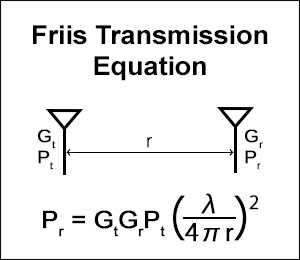# Friis Transmission Calculator

This calculator calculates the received power of an antenna given from the distance, antenna gains, frequency/wavelength, and transmitting antenna power.

The calculator uses one form of the Friis Transmission Equation to calculate that.

The "(λ/4*π*r)^2" inside the equation is equal to the inverse function of free space path loss.

The output values are rounded to the second decimal point. The speed of light is calculated as 299792458(m/s)(Only used when a frequency value is given).

One form of the Friis Transmission Equation

Pr = Gt*Gr*Pt*(λ/4*π*r)^2

λ= Wavelength(m)、r = Distance(m)、Pt = Transmitting Antenna Power(W)、Gt = Transmitting Antenna Gain(linear)、Pr = Receiving Antenna Power(W)、Gr = Receiving Antenna Gain(linear)### Input

r:

f:

λ:

Pt: W

Gt: times

Gr: times
Use frequency value
Use wavelength value